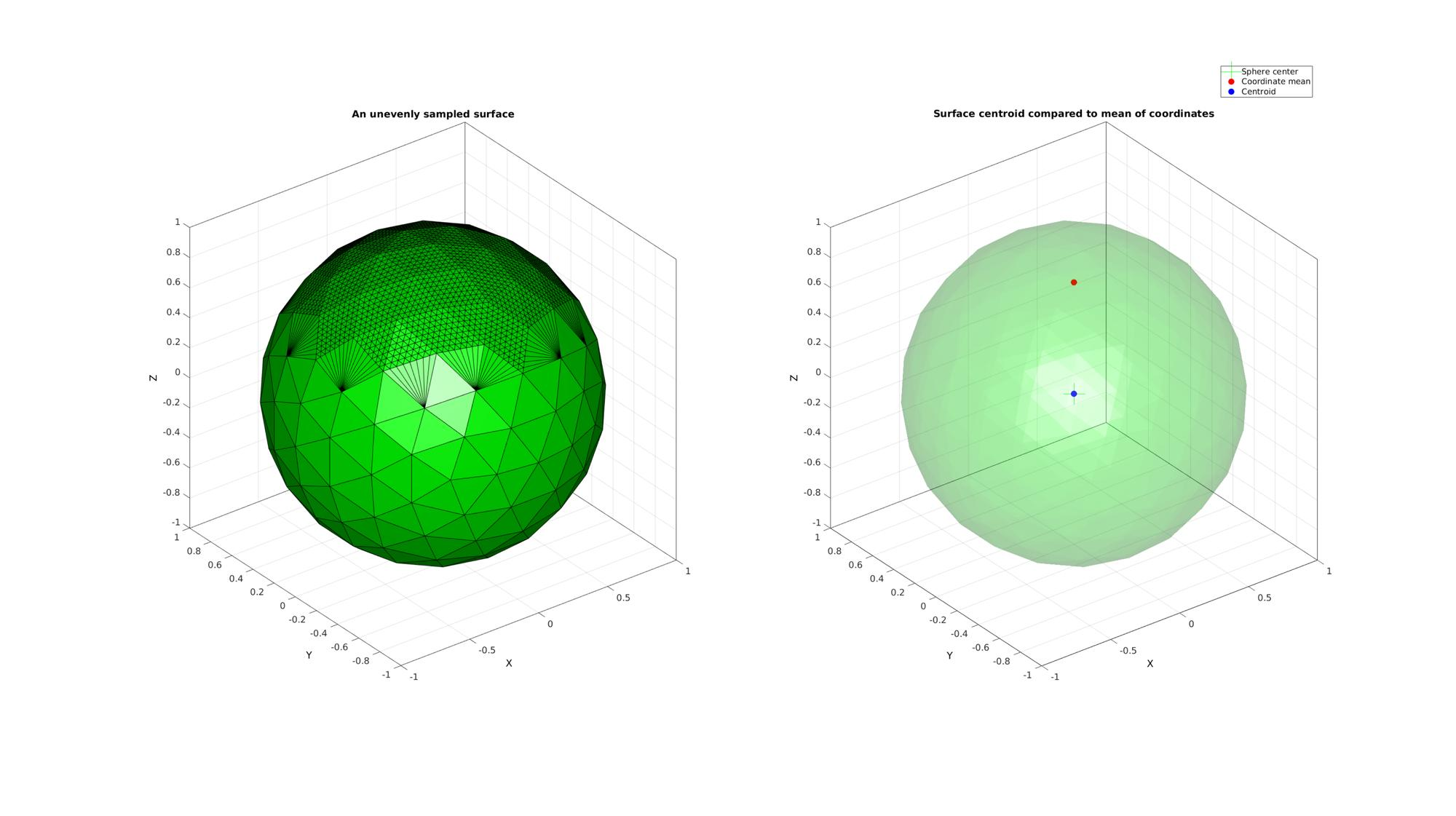## Contents

```clear; close all; clc;
```

## triSurfCentroid

Below is a demonstration of the features of the triSurfCentroid function

## Syntax

[Vc]=triSurfCentroid(F,V);

## Description

The triSurfCentroid function enables refinement of triangulated data

## Examples

```clear; close all; clc;
```

Plot Settings

```fontSize=15;
faceAlpha=0.2;
markerSize=35;
```

## Example: Calculating the centriod of a triangulated surface

Building example geometry, in this case a sphere

```%Defining geodesic dome
n=2; %Refinements
[F,V,~]=geoSphere(n,r);
```

The geoSphere function creates a homogeneously sampled sphere, hence the mean of the coordinates coincides with the centroid. To see the difference with the centroid we'd need to change the sampling.

```% Using |subTriLocal| to refine a top region of the sphere which will
% offset the mean of the coordinates from the centroid.

nRefine=3; %Number of local refinement steps
for q=1:1:nRefine
%Create logic for faces to refine
L=V(:,3)>0.5;
LF=all(L(F),2);
indFaces=find(LF);

%Refining the surface locally
inputStruct.F=F;
inputStruct.V=V;
inputStruct.indFaces=indFaces;
[outputStruct]=subTriLocal(inputStruct);
F=outputStruct.F;
V=outputStruct.V;
end
```

Now the mean of the coordinates and the centroid will be calculated.

```Vm=mean(V,1); %Mean of the coordinates

[Vc]=triSurfCentroid(F,V); %Centroid of the surface
```

Plotting results

```cFigure;
subplot(1,2,1); hold on;
title('An unevenly sampled surface','fontSize',fontSize);
gpatch(F,V,'g','k',1);
axisGeom(gca,fontSize);

subplot(1,2,2); hold on;
title('Surface centroid compared to mean of coordinates','fontSize',fontSize);
gpatch(F,V,'g','none',faceAlpha);
h1=plotV([0 0 0],'g+','MarkerSize',markerSize);
h2=plotV(Vm,'r.','MarkerSize',markerSize);
h3=plotV(Vc,'b.','MarkerSize',markerSize);

legend([h1 h2 h3],'Sphere center','Coordinate mean','Centroid');

axisGeom(gca,fontSize);
drawnow;
```GIBBON www.gibboncode.org

Kevin Mattheus Moerman, [email protected]

GIBBON footer text

GIBBON: The Geometry and Image-based Bioengineering add-On. A toolbox for image segmentation, image-based modeling, meshing, and finite element analysis.

Copyright (C) 2019 Kevin Mattheus Moerman

This program is free software: you can redistribute it and/or modify it under the terms of the GNU General Public License as published by the Free Software Foundation, either version 3 of the License, or (at your option) any later version.

This program is distributed in the hope that it will be useful, but WITHOUT ANY WARRANTY; without even the implied warranty of MERCHANTABILITY or FITNESS FOR A PARTICULAR PURPOSE. See the GNU General Public License for more details.

You should have received a copy of the GNU General Public License along with this program. If not, see http://www.gnu.org/licenses/.